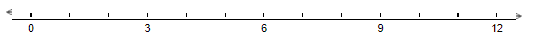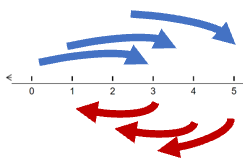# Math Worksheets Land

Math Worksheets For All Ages

# Math Worksheets Land

Math Worksheets For All Ages

Home > Math Topics > Patterns & Sequence >

# Math Patterns on Number Lines Worksheets

Number lines are great tools for visualizing numbers, operations, and data sets to life. When they are featured in this form, it makes it easy to spot trends and see if anything exists that may have been overlooked. When you view data in this format it makes it much easier to get to the bottom of any trends or patterns that may exist within it. Students will use a number line to discover patterns in number sequences with these worksheets and lessons. It is a good habit to get into.

### Aligned Standard: 6.SP.B.4

• Answer Keys - These are for all the unlocked materials above.

### Showing Patterns Sheets

What does each twist and turn mean on the grid.

• Homework 1 - Follow the pattern rule to draw hops on each number line.
• Homework 2 - How many arrows did you draw?
• Homework 3 - Find where the pattern starts.

### Practice Worksheets

It is best if you write these out in words and sentences.

• Practice 2 - Tell about the pattern that you see.
• Practice 3 - Since the pattern completes all intervals, we do not need to worry about where the pattern ends.

### Math Skill Quizzes

The first two ask you to interpret patterns and the last one asks you to make one.

• Quiz 1 - Look at where the arrows point and determine the interval of the pattern.
• Quiz 2 - Put those statements together to make a statement that someone could use to create this pattern.
• Quiz 3 - We can now see where the pattern starts and stops. We can see that the pattern goes right to left.

### How to Show Math Patterns on Number Lines

The number line in mathematics is used for many purposes. In some instances, it is used simply for representing numbers on a line. It can also be used to display operations that exist in an equation. Common examples of number lines include rulers, measuring scales, analog clocks, graph axes, timelines, and analogue clocks. All these are examples of number lines. A number line can be both curved and a straight line. The curved form usually is only used when you have very few segments because the curve helps spread them out and the elevation allows you to clearly delineate between all the different values. Want to learn how you can show math patterns on a number line? Let's find out.

Counting - You can link the number line with counting. Create a pattern that will skip counting or even a straight count that can begin at any value of your choice. Represent it on a number line in a way that the pattern repeats. For example;

1, 2, 3, 4, 5, 6, 7, 8, 9, 10, 11, 12, 13, 14, 15, 16, 17, 18, 19, 200 to 12 Number Line

Skip Counting - The above number line shows a pattern that represents the table of 3. The equal distance and spacing on the number line helps students to learn skip counting. Similarly, you can represent another pattern on the number line. 4, 7, 12, 13, 16, 19, 22, 25, 28, 31; the pattern here is adding 1,2,3,4 starting from 12 to 31. The pattern starts with 12, and then you add 1 you get 13, then you add 2 you get 2, then you add 3 you get 19 and this continues till the end of the number line. The ONLY key is to IDENTIFY that there is a pattern.

Jumping Around - Some patterns are based on fixed rules that may go forward or backward. In some cases, they can do both. If you play board games as a kid, you might remember taking three steps forward and two backward. The same can be true in a pattern. We can visualize the three steps forward and two back in a series, just check out this number line:Unlock all the answers, worksheets, homework, tests and more!
Save Tons of Time! Make My Life Easier Now

## Thanks and Don't Forget To Tell Your Friends!

I would appreciate everyone letting me know if you find any errors. I'm getting a little older these days and my eyes are going. Please contact me, to let me know. I'll fix it ASAP.